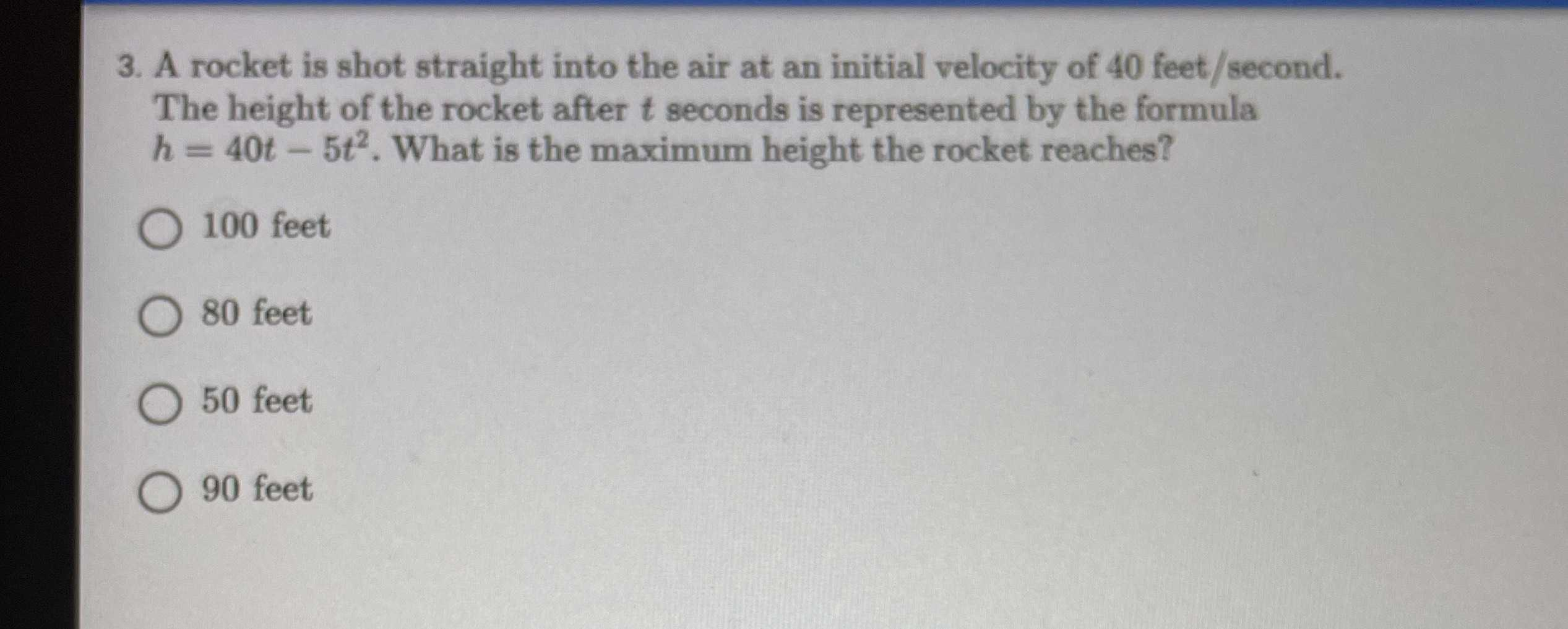### ¿Todavía tienes preguntas de matemáticas?

Pregunte a nuestros tutores expertos
Algebra
Pregunta3. A rocket is shot straight into the air at an initial velocity of $$40$$ feet/second. The height of the rocket after $$t$$ seconds is represented by the formula

$$h = 40 t - 5 t ^ { 2 }$$ . What is the maximum height the rocket reaches?

$$100$$ feet

$$80$$ feet

$$50$$ feet

$$90$$ feet